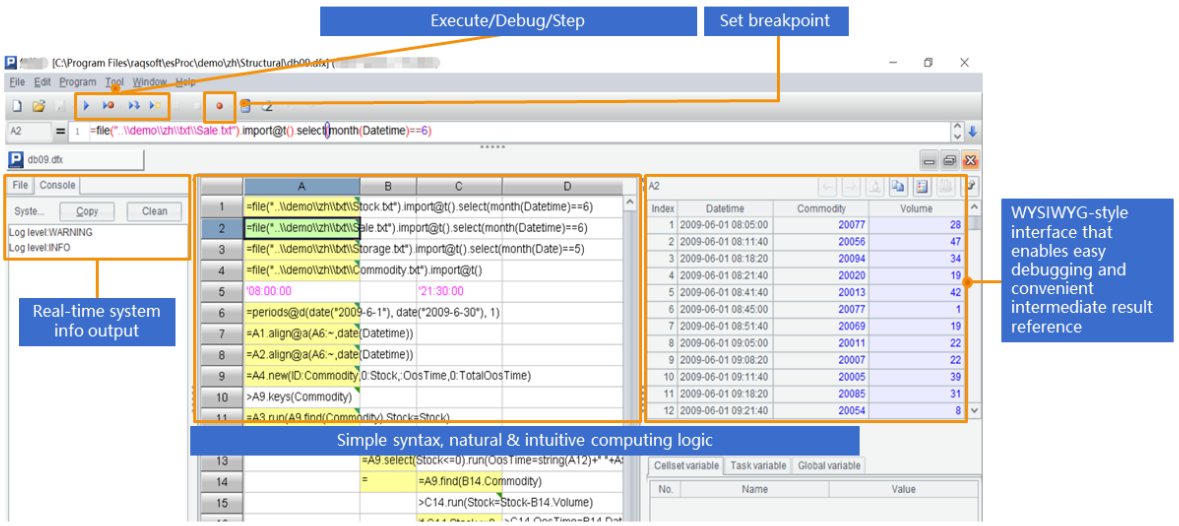# Java 中如何查询计算 xls？

POI可以解析xls，但不提供进一步查询计算的能力，取出数据后都要自己硬写。内嵌数据库可以查询计算，但又不能解析，而且入库过程非常耗时，架构又臃肿得很。直接在xls上执行SQL是个快速轻便的好方法，但相关的开源库很少，现在都还只是雏形，实用价值不高。DataFrame类的开源库省去了解释SQL的麻烦，完成度高一些，但同时也失去了松耦合的可能性，而且计算能力远不如SQL。

SPL提供了简单易用的解析函数，可以轻松读取xls。比如，Excel文件的每行是一条订单记录，首行是列名，将该文件解析为二维表，只需简单写作：

`=T("D:/data.xls")`

```=file("D:/data.xlsx").xlsimport@t(;"Orders")
```

SPL提供了丰富的库函数，可直接完成常见的计算：

```//条件查询：
=T("D:/Orders.xls").select(Amount>1000   && Amount<=3000 && like(Client,"*S*"))
//排序
=T("D:/Orders.xls").sort(Client,-Amount)";
//去重
=T("D:/Orders.xls").id(Client)";
//分组汇总
=T("D:/Orders.xls").groups(year(OrderDate);sum(Amount))";
//xlsx关联txt
=join(T("D:/Orders.xlsx"):O,SellerId; T("D:/data/Employees.txt"):E,EId).new(O.OrderID,O.Client,O.SellerId,O.Amount,O.OrderDate,   E.Name,E.Gender,E.Dept)";
```

SPL提供了JDBC接口，JAVA代码可轻松调用，以条件查询为例：

```…
Class.forName("com.esproc.jdbc.InternalDriver");
Connection connection   =DriverManager.getConnection("jdbc:esproc:local://");
Statement statement = connection.createStatement();String str="=T(\"D:/Orders.xls\").select(Amount>1000   && Amount<=3000 && like(Client,\"*S*\"))";
ResultSet result = statement.executeQuery(str);
…```

```str="\$select   * from d:/Orders.xls where Client like'%S%'or (Amount>1000 and   Amount<=2000)"
```

SPL支持计算代码外置，修改计算代码时无需编译，适合较复杂或频繁修改的计算，可彻底降低耦合性。做为对比，内嵌库通常不支持存储过程，而大型数据库的存储过程需要编译。这里试举一例，在各部门找出比本部门平均年龄小的员工。先将SPL算法存为脚本文件：

 A 1 =T("Employee.xls") 2 =A1.group(DEPT; (a=~.avg(age(BIRTHDAY)),~.select(age(BIRTHDAY)

```…
Class.forName("com.esproc.jdbc.InternalDriver");
Connection connection   =DriverManager.getConnection("jdbc:esproc:local://");
Statement statement =   connection.createStatement();

ResultSet result =   statement.executeQuery("call getYoung()");
...```

 A 1 =file("D:/data/keyvalue.xlsx").xlsimport@w() 2 =A1.conj().(~.split("=")) 3 =A2.new(~(1),~(2))

 A 1 =T("d:/AAPL.xlsx") 2 =a=0,A1.max(a=if(price>price[-1],a+1,0))

SPL提供了专业的IDE，适合设计逻辑复杂的计算，不仅具备完整的调试功能，还可以观察每一步的计算结果。